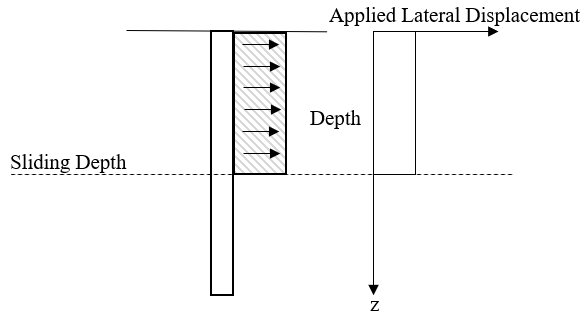# Displacement

The Displacement tab houses the Displacement Profile and Soil Displacement features. The options can only be used independently of each other for both lateral and axial analysis.

### DISPLACEMENT PROFILE

In the Edit Displacement Profiles dialog, accessed by clicking on the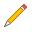icon beside the Profile dropdown, Displacement Profiles can be created and edited.

As mentioned above, when this option is enabled, the Soil Displacement option cannot be used and the analysis will run based on the displacement profile. To simulate a displacement profile combined with a sliding depth, put zeros below the sliding depth location - below the sliding depth, there is no soil movement.

This user-defined Displacement Profile isn't used in Slide and Slide analyses assumes a uniform soil displacement. The only option for generating the Lateral Resistance Graph and Axial Resistance Graph is the Max Allowable Lateral Displacement and Max Allowable Axial Displacement options, respectively.

See Computing Pile Resistance from Applied Soil Displacement for Slope Stability Analysis for more information on this topic.

The user has the option to enter a soil displacement profile down the axis of the pile that moves perpendicular to the pile axis. X or Y displacements can be added.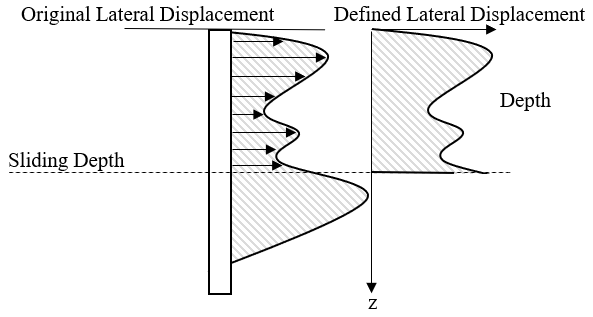General lateral displacement profile to calculate lateral resistance

The user can enter the axial soil displacement at various depths along the pile axis. Note that the soil displacement and pile depth are measured along the pile axis, which may be rotated due to the batter angle.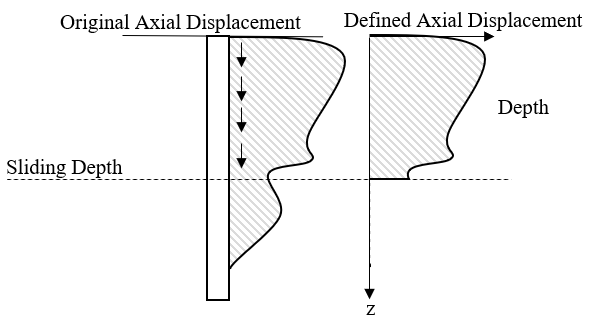General axial displacement profile to calculate axial resistance

### SOIL DISPLACEMENT

In the Soil Displacement section, the user has the following two choices:

• Soil Displacement and Sliding Depth - lateral/axial soil loading is defined using the automated uniform soil displacement profile
• Lateral / Axial Resistance Graph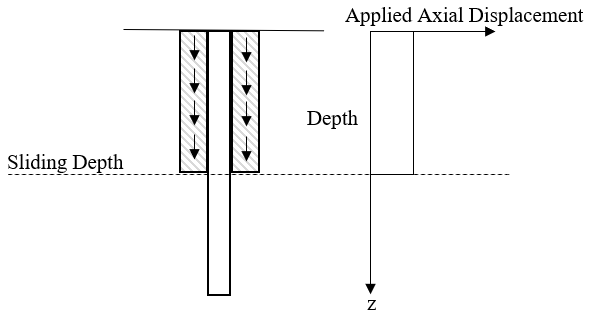Automated uniform axial displacement profile